# Knowing that a = 21°, determine the resultant of the three forces shown. 100 N 200...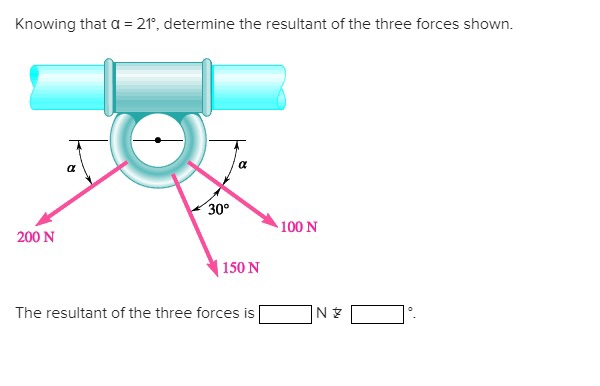Knowing that a = 21°, determine the resultant of the three forces shown. 100 N 200 N 150 N The resultant of the three forces is NEO

Hi dear.

i have solved your queation and attaching the solution in picture form.

please give postive feedback if you are satisfied with my solution approch.

thank you.

.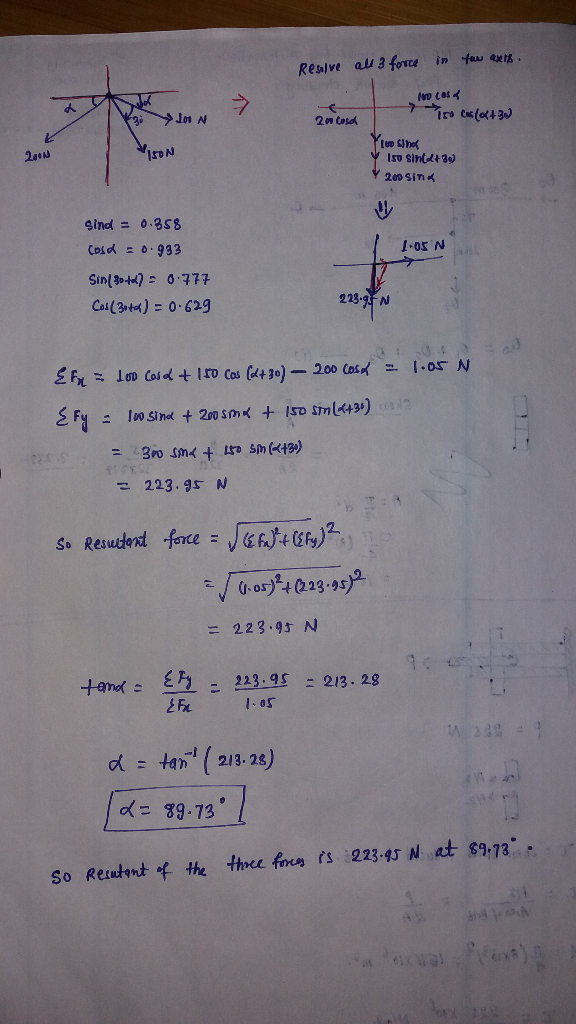##### Add Answer to: Knowing that a = 21°, determine the resultant of the three forces shown. 100 N 200...
Similar Homework Help Questions
• ### Knowing that the tension in cable BCis 760 N, determine the resultant of the three forces...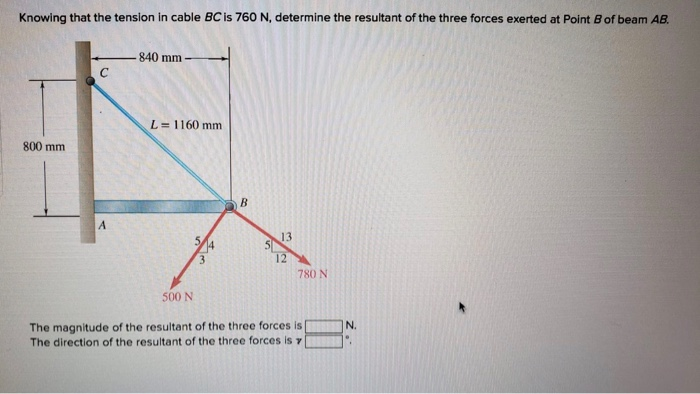Knowing that the tension in cable BCis 760 N, determine the resultant of the three forces exerted at Point B of beam AB 840 mm- L= 1160 mm 800 mm 13 12 780 N 500 N The magnitude of the resultant of the three forces is The direction of the resultant of the three forces is γ N.

• ### F-100 N ON 8 ne the resultant of the three forces showr s of i. J and k 96.6 N + 1 90,7 NJ-100 N k) b09 F: - 200 N F3-300 N 3-2 am a Question Determine the resultant of the three forces sho...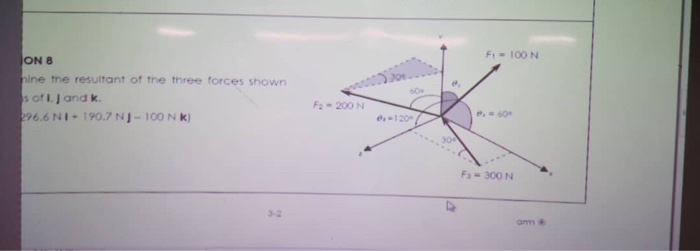F-100 N ON 8 ne the resultant of the three forces showr s of i. J and k 96.6 N + 1 90,7 NJ-100 N k) b09 F: - 200 N F3-300 N 3-2 am a Question Determine the resultant of the three forces shown in terms of i, j, and k. F-100 N ON 8 ne the resultant of the three forces showr s of i. J and k 96.6 N + 1 90,7 NJ-100 N k) b09 F:...

• ### How big is the resultant of the three forces?

Three athletes are tugging on a ring. Martha pulls with a force of 150 N in the northerly direction. Eveylyn pulls with a force of 100 N in the easterly direction.Sara pulls with a force of 200 N in the southwesterly direction (225 degrees clockwise from north).a) How big is the resultant of the three forces?b) What is the direction of the resultant force?

• ### Determine the resultant (magnitude and direction of the three forces shown. All forces are in kips....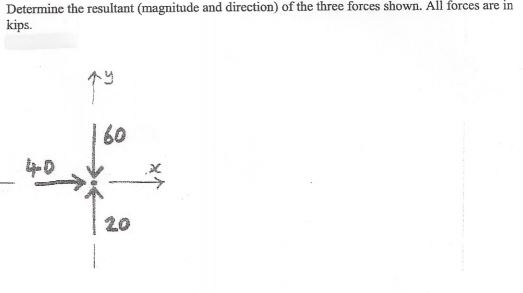Determine the resultant (magnitude and direction of the three forces shown. All forces are in kips. q 60 -- 20

• ### 1.2 Determine the resultant (magnitude and direction) of the three forces shown using the graphical method....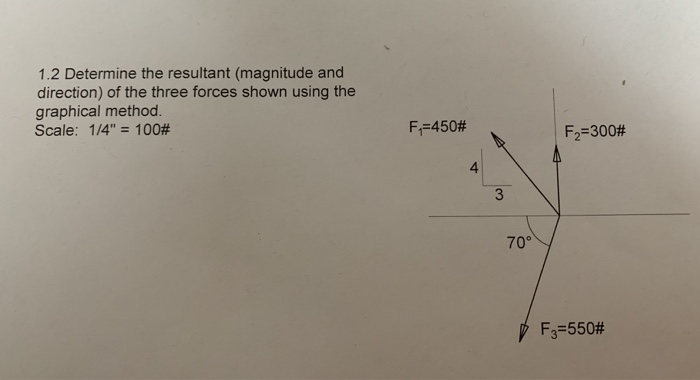1.2 Determine the resultant (magnitude and direction) of the three forces shown using the graphical method. Scale: 14"-100# FF450# F-300# 4 70° F3-550#

• ### 500 N C 25 24 Knowing that the tension in rope AC is 365 N determine...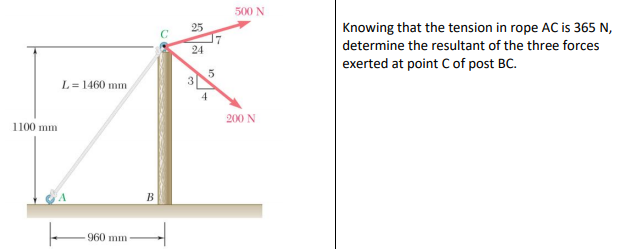500 N C 25 24 Knowing that the tension in rope AC is 365 N determine the resultant of the three forces exerted at point C of post BC L = 1460 mim 3 200 N 1100 mm 960 mm ının

• ### 1. Two forces are applied as shown to a hook support. Knowing that the magnitude of...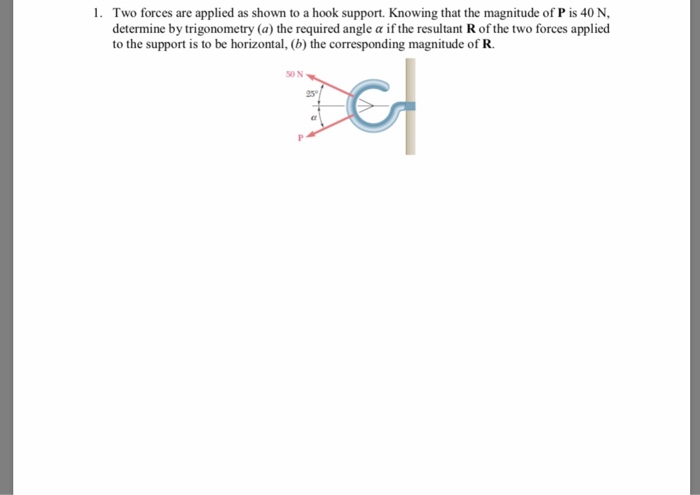1. Two forces are applied as shown to a hook support. Knowing that the magnitude of P is 40 N determine by trigonometry (a) the required angle a if the resultant R of the two forces applied to the support is to be horizontal, (b the corresponding magnitude of R. 50 N 25

• ### 2.10 Two forces are applied as shown to a hook support. Knowing that the magnitude of...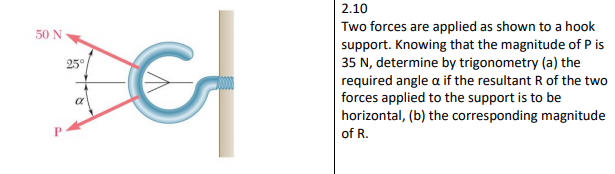2.10 Two forces are applied as shown to a hook support. Knowing that the magnitude of P is 35 N, determine by trigonometry (a) the required angle α if the resultant R of the two forces applied to the support is to be horizontal, (b) the corresponding magnitude of R. 50 N 250%

• ### We were unable to transcribe this image2.43 Determine the resultant of the three forces shown acting...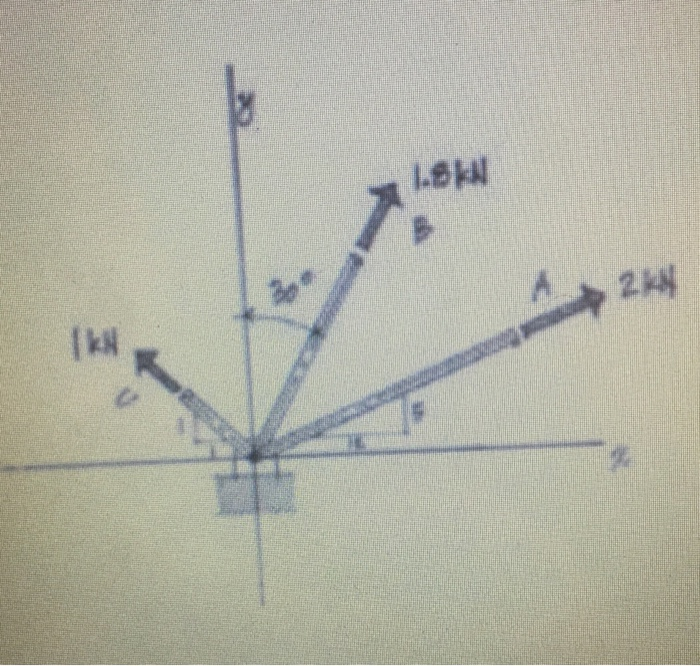We were unable to transcribe this image2.43 Determine the resultant of the three forces shown acting on the anchor device. Use the tip-to-tail graphical method in your solution. Follow a sequence of A-B-C. Scale: 50 mm 1 kN or 1 mm 20 N.

• ### P.2 Two forces are applied as shown to a hook support. Knowing that the magnitude of...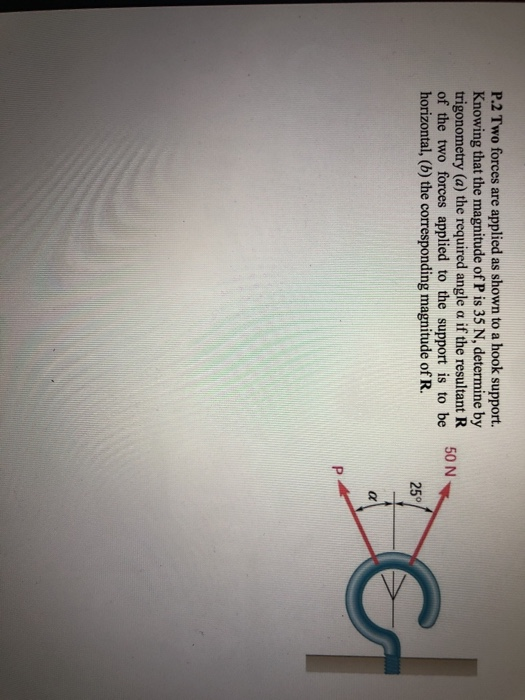P.2 Two forces are applied as shown to a hook support. Knowing that the magnitude of P is 35 N, determine by trigonometry (a) the required angle a if the resultant R of the two forces applied to the support is to be horizontal, (b) the corresponding magnitude of R. Cs 25°

Free Homework App